# S Fartoukh D Pellegrini Contents The starting point

• Slides: 21
Download presentation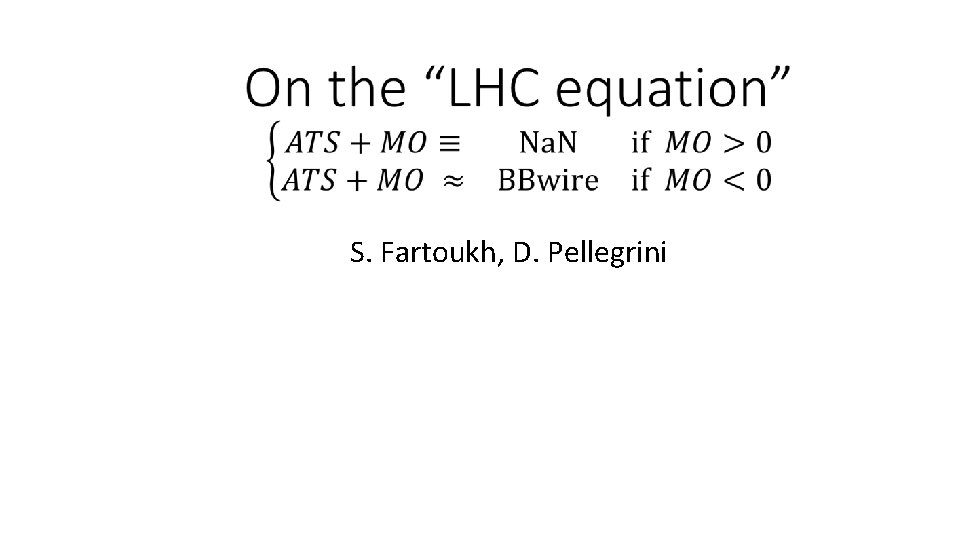S. Fartoukh, D. Pellegrini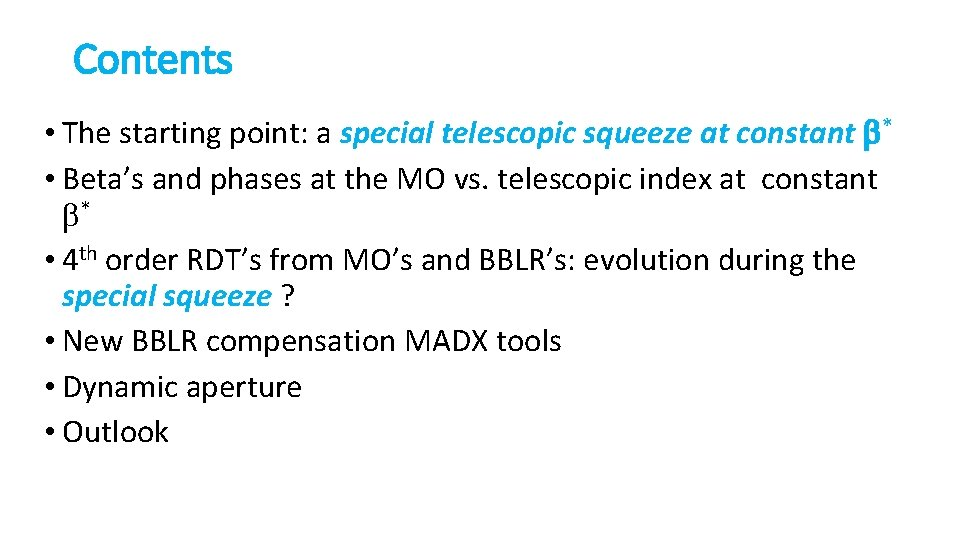Contents • The starting point: a special telescopic squeeze at constant b* • Beta’s and phases at the MO vs. telescopic index at constant b* • 4 th order RDT’s from MO’s and BBLR’s: evolution during the special squeeze ? • New BBLR compensation MADX tools • Dynamic aperture • Outlook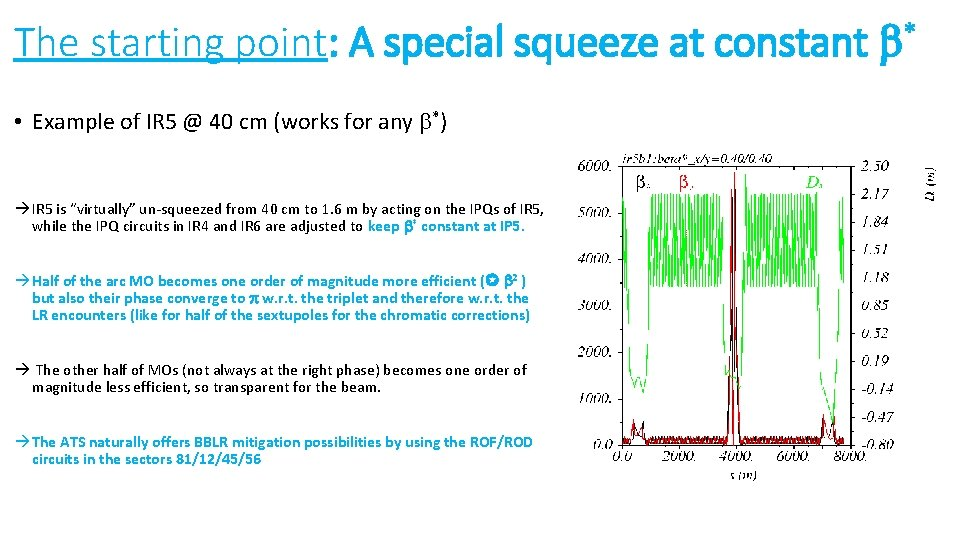The starting point: A special squeeze at constant b* • Example of IR 5 @ 40 cm (works for any b*) IR 5 is “virtually” un-squeezed from 40 cm to 1. 6 m by acting on the IPQs of IR 5, while the IPQ circuits in IR 4 and IR 6 are adjusted to keep b* constant at IP 5. Half of the arc MO becomes one order of magnitude more efficient ( b 2 ) but also their phase converge to p w. r. t. the triplet and therefore w. r. t. the LR encounters (like for half of the sextupoles for the chromatic corrections) The other half of MOs (not always at the right phase) becomes one order of magnitude less efficient, so transparent for the beam. The ATS naturally offers BBLR mitigation possibilities by using the ROF/ROD circuits in the sectors 81/12/45/56b’s and phases at the MO’s vs. telescopic index (1/3) • Two categories of octupoles in s 81/12/45/56: the “strong” (with increased b 1/b*) and the “weak” (with decreased b b*) Strong OD Strong OF Weak OF Telescopic squeeze (× 4 x, × 4 y) Beam 1/2 Weak OD “Strong“ OF. b 1/OD. b 2 Weak OF. b 1/OD. b 2 Strong OD. b 1/OF. b 2 Weak OD. b 1/OF. b 2 Sector 45 (81) 22. R 4(8), 26. R 4(8), 30. R 4(8), 34. R 4(8), 30. L 5(1), 26. L 5(1), 22. L 5(1) 24. R 4(8), 28. R 4(8), 32. R 4(8), 32. L 5(1), 28. L 5(1), 24. L 5(1) 25. R 4(8), 29. R 4(8), 33. R 4(8), 31. L 5(1) 31. R 4(8), 33. L 5(1), 29. L 5(1), 25. L 5(1) Sector 56 (12) 31. R 5(1), 33. L 6(2), 29. L 6(2), 25. L 6(2) 25. R 5(1), 29. R 5(1), 33. R 5(1), 31. L 6(2) 22. R 5(1), 26. R 5(1), 30. R 5(1), 34. R 5(1), 30. L 6(2), 26. L 6(2), 22. L 6(2) 24. R 5(1), 28. R 5(1), 32. R 5(1), 32. L 6(2), 28. L 6(2), 24. L 6(2) 7+4 = 11 6+4 =10 4+7 =11 4+6=10 Totalb’s and phases at the MO’s for telescopic optics (2/3) • As for the strong sextupoles (participating to the IT chromatic correction), all the strong OF (resp. OD) are located at an H phase (resp. V phase) very close to p/2+ kp w. r. t. the IP, so p w. r. t. the triplet and the LR beam-beam encounters Telescopic squeezeb’s and phases at the MO’s for telescopic optics (3/3) • Contrary to the pre-squeezed optics, only close to p/2 and p phases w. r. t the triplet are present for telescopic optics (phases like p/4+kp are no longer seen by octupole) A clean compensation of the LR beam-beam effects is a priori within reach for both the octupole tune spread and the (4, 0), (0, 4) and (2, ± 2) driving terms Telescopic squeeze (× 4 x, × 4 y) p/2 + e p/2 - e4 th order driving terms and tune spread (1/4) • From octupoles For IP 1 (resp. IP 5) the relevant figures of merit of a given telescopic optics is given by the following normalized driving terms C(p, q), (p, q)= (4, 0), (0, 4), (2, 2) or (2, -2), rephased w. r. t. the IP: CMO-norm. (p, q) 1 means that tune spread and driving terms correspond to the same quantity as for the octupolar part of the LR beam-beam effects (see next). CMO-norm. (p, q) real (< 1 by construction) means that the driving terms only correspond to a fraction of the induced tune spread, but are in phase w. r. t the LR beam-beam induced 4 th driving terms The efficiency of the octupoles (i. e. the non-normalized coefficient |CMO (pq)|) obviously increases during the telescopic squeeze4 th order driving terms and tune spread (2/4) (0, 4) Phase [ deg. ] w. r. t. the IP Ratio over tune spread • From octupoles (cont’d) (4, 0) Telescopic squeeze The larger is the telescopic index, the better is the coincidence between the direct anharmonicity coefficients and the (4, 0) and (0, 4) driving terms.4 th order driving terms and tune spread (3/4) (2, -2) Phase [ deg. ] w. r. t. the IP Ratio over tune spread • From octupoles (cont’d) (2, 2) Telescopic squeeze For the pre-squeezed optics the (2, 2) RDT is zero (p/4 between consecutive octupole) For the telescopic 44 optics, 75% of the X-anharmonicity is reached, the (2, 2) RDT phase w. r. t. the IP is almost 0 degrees ! For the pre-squeezed optics the (2, -2) RDT correspond to 100% of the X-anharmonicity but is in quadrature of phase For the telescopic 44 optics, 80 -85% of the X-anharmonicity is kept, and the phase reduces to 40 degrees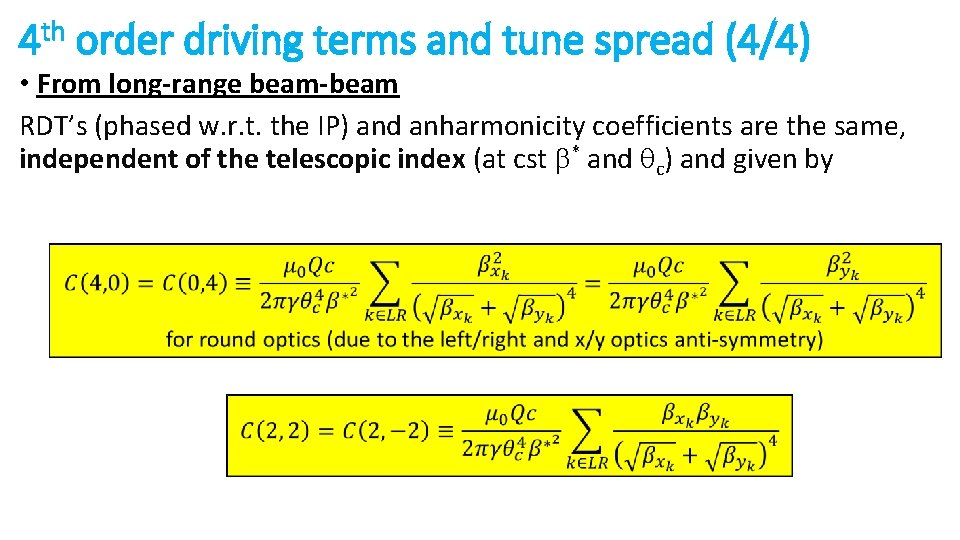4 th order driving terms and tune spread (4/4) • From long-range beam-beam RDT’s (phased w. r. t. the IP) and anharmonicity coefficients are the same, independent of the telescopic index (at cst b* and qc) and given byNew MADX LR correction tool with octupoles (1/7) • The problem (for “standard telescopic round optics): optics) LR and MO anharmonicity matrices are NOT collinear. . Example: LRs in IR 1/5 only, Nb=1. 20 E 11, ge=2. 5 mm, qx=+/- 140 mrad, b*=40 cm Particles in the V plane Particles in the H plane Particles at 45 degrees Particles in the H plane LR only: Qx/d. Jx = Qy/d. Jy ~ - Qx/d. Jy Very thin extension on the diagonal Particles in the V plane MO only (LOF <0): Qx/d. Jx = Qy/d. Jy >> Qx/d. Jy è Rather thick extension on the diagonal adding with the LRs è Counter-acting the LRs on the anti-diagonal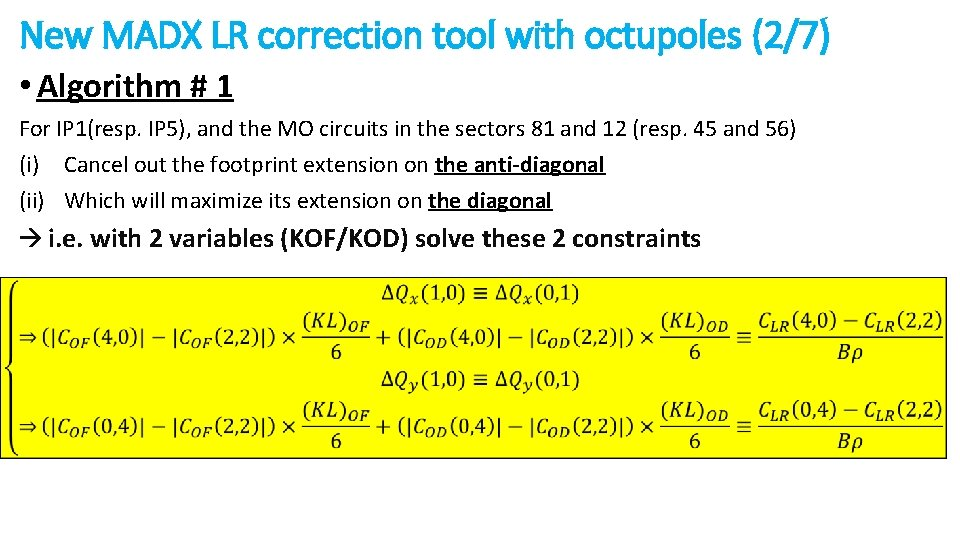New MADX LR correction tool with octupoles (2/7) • Algorithm # 1 For IP 1(resp. IP 5), and the MO circuits in the sectors 81 and 12 (resp. 45 and 56) (i) Cancel out the footprint extension on the anti-diagonal (ii) Which will maximize its extension on the diagonal i. e. with 2 variables (KOF/KOD) solve these 2 constraints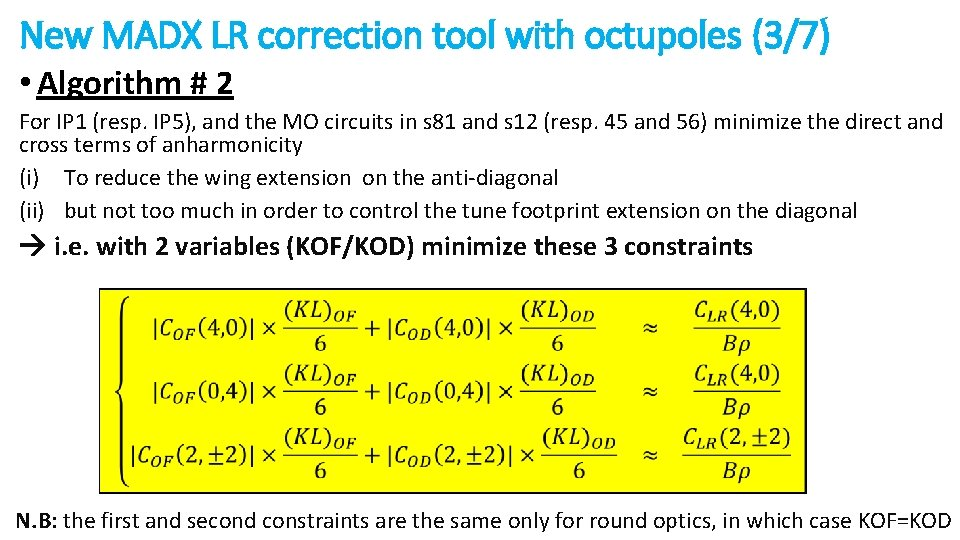New MADX LR correction tool with octupoles (3/7) • Algorithm # 2 For IP 1 (resp. IP 5), and the MO circuits in s 81 and s 12 (resp. 45 and 56) minimize the direct and cross terms of anharmonicity (i) To reduce the wing extension on the anti-diagonal (ii) but not too much in order to control the tune footprint extension on the diagonal i. e. with 2 variables (KOF/KOD) minimize these 3 constraints N. B: the first and second constraints are the same only for round optics, in which case KOF=KOD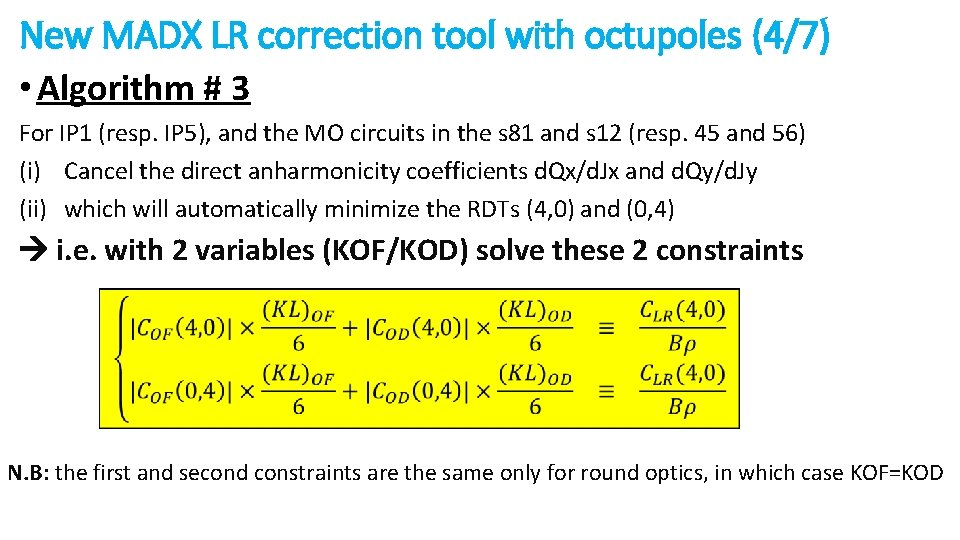New MADX LR correction tool with octupoles (4/7) • Algorithm # 3 For IP 1 (resp. IP 5), and the MO circuits in the s 81 and s 12 (resp. 45 and 56) (i) Cancel the direct anharmonicity coefficients d. Qx/d. Jx and d. Qy/d. Jy (ii) which will automatically minimize the RDTs (4, 0) and (0, 4) i. e. with 2 variables (KOF/KOD) solve these 2 constraints N. B: the first and second constraints are the same only for round optics, in which case KOF=KODNew MADX LR correction tool with octupoles (5/7) • Example with a telescope index of 4 (peak b × 4 in the arcs) Footprint at 5 s w/o head-on : LRs in IR 1/5, N =1. 20 E 11, ge=2. 5 mm, q =+/- 140 mrad, b =40 cm b x * (no correction) Algorithm #1 giving IMO=- 450 A Algorithm #2 giving IMO=- 300 A Algorithm #3 giving IMO=- 230 A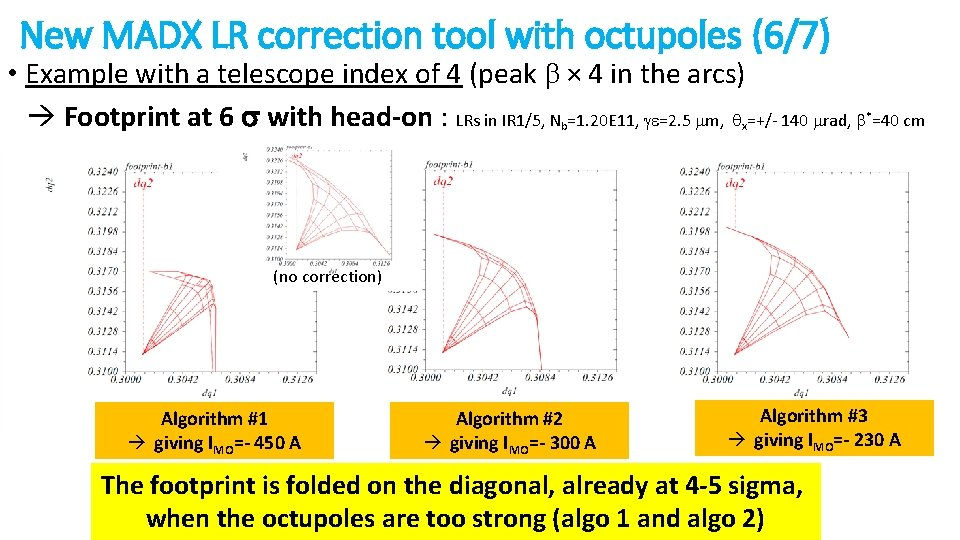New MADX LR correction tool with octupoles (6/7) • Example with a telescope index of 4 (peak b × 4 in the arcs) Footprint at 6 s with head-on : LRs in IR 1/5, N =1. 20 E 11, ge=2. 5 mm, q =+/- 140 mrad, b =40 cm b x * (no correction) Algorithm #1 giving IMO=- 450 A Algorithm #2 giving IMO=- 300 A Algorithm #3 giving IMO=- 230 A The footprint is folded on the diagonal, already at 4 -5 sigma, when the octupoles are too strong (algo 1 and algo 2)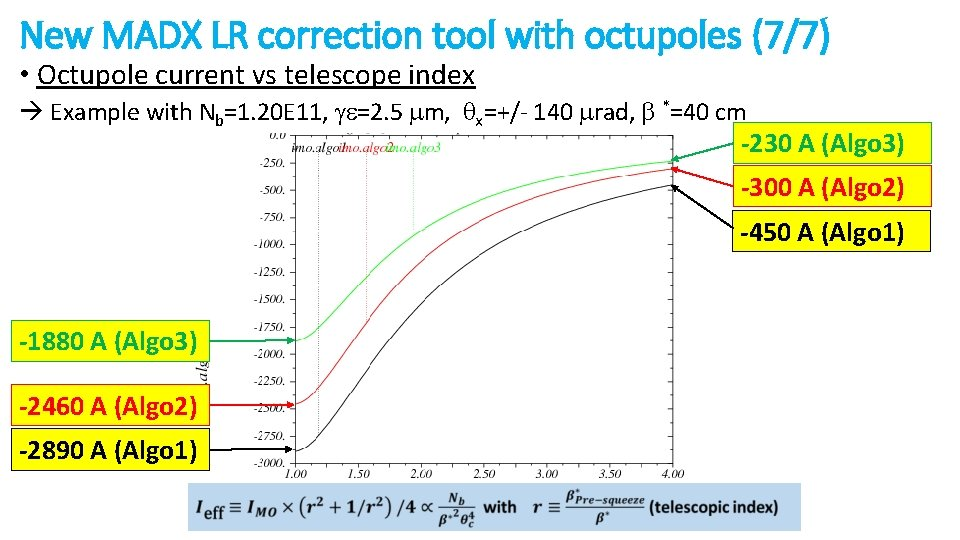New MADX LR correction tool with octupoles (7/7) • Octupole current vs telescope index Example with Nb=1. 20 E 11, ge=2. 5 mm, qx=+/- 140 mrad, b *=40 cm -230 A (Algo 3) -300 A (Algo 2) -450 A (Algo 1) -1880 A (Algo 3) -2460 A (Algo 2) -2890 A (Algo 1)Dynamic aperture results (1/2) • Residual driving terms and detunings as a function of the MO current Example: Nb=1. 20 E 11, ge=2. 5 mm, qx=+/- 140 mrad, b*=40 cm Algo 3 Not satisfactory for (2, -2) but with large telescope index (r ~ 4) and [email protected] -500 A (<0), all other quantities can be decreased by ~ 50%Dynamic aperture results (2/2) Courtesy of D. Pellegrini Ieff ~ 1 k. A is optimal, i. e. IMO~ 2 k. A for r=1 , IMO~ 600 A for r=2. 5 and IMO ~ 250 A for r=4 This corresponds to Algo 3 ((4, 0) and (0, 4) RDT & direct anharmonicity)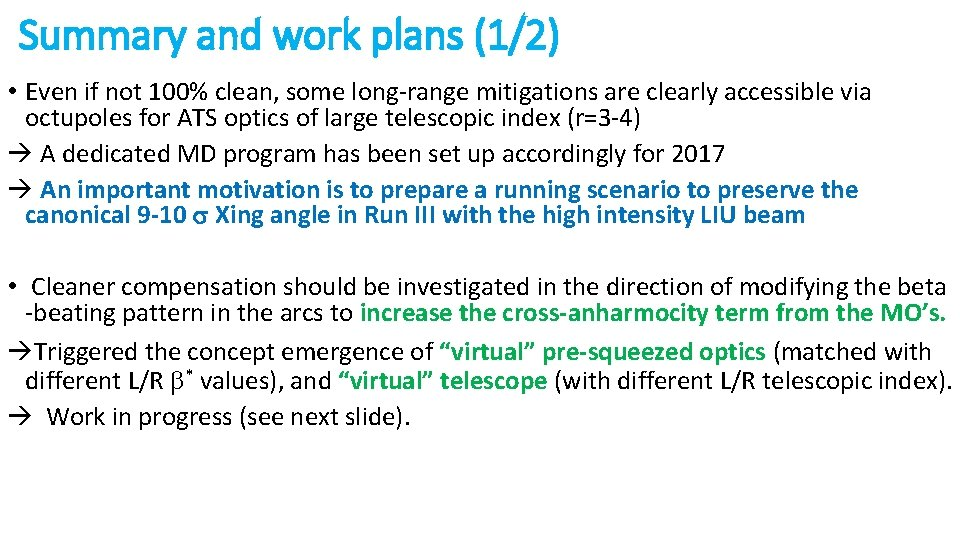Summary and work plans (1/2) • Even if not 100% clean, some long-range mitigations are clearly accessible via octupoles for ATS optics of large telescopic index (r=3 -4) A dedicated MD program has been set up accordingly for 2017 An important motivation is to prepare a running scenario to preserve the canonical 9 -10 s Xing angle in Run III with the high intensity LIU beam • Cleaner compensation should be investigated in the direction of modifying the beta -beating pattern in the arcs to increase the cross-anharmocity term from the MO’s. Triggered the concept emergence of “virtual” pre-squeezed optics (matched with different L/R b* values), and “virtual” telescope (with different L/R telescopic index). Work in progress (see next slide).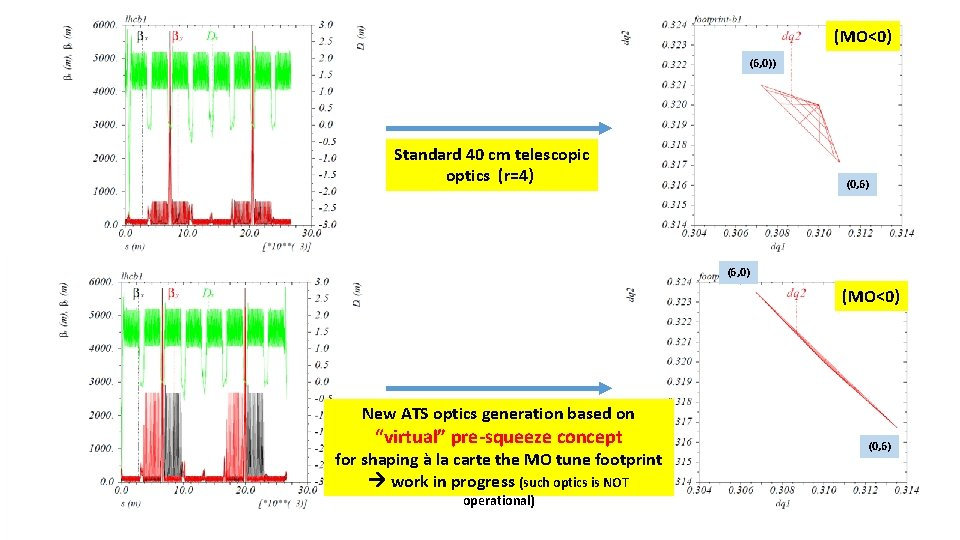(MO<0) (6, 0)) Standard 40 cm telescopic optics (r=4) (0, 6) (6, 0) (MO<0) New ATS optics generation based on “virtual” pre-squeeze concept for shaping à la carte the MO tune footprint work in progress (such optics is NOT operational) (0, 6)